Slopes and Intercepts in Linear Models MathBitsNotebook.com Terms of Use   Contact Person: Donna RobertsLinear regression equations are predictors, or indicators, of trends in the data. Let's see what we can find out about the data relationships by examining linear regressions.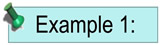Data was collected regarding the 15 team members on the Jackson High School Baseball Team. The number of times each player visited the batting cage in the 3 months prior to the start of the season, along with each player's season batting average, were recorded (as seen in the table at the right).

Is this a linear regression?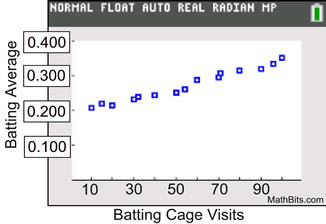We make a scatter plot, and it appears that the plots are rising from left to right, and are clustered around a possible straight line.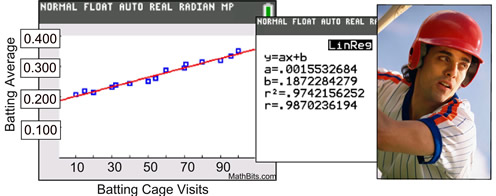Batting Cage Visits Pre-season Season Batting Average 10 .208 15 .220 20 .215 30 .231 32 .238 40 .245 50 .250 54 .261 60 .289 70 .294 71 .308 80 .315 90 .320 96 .335 100 .350
The calculator prepared a linear regression
on the data and arrived at the
linear regression equation y = 0.002x + 0.187
(rounded to nearest thousandths).
(The residual scatter plot, at the right, shows that a linear model is
appropriate for this data, since the pattern is random.)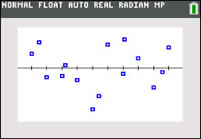What is the slope of the linear regression line, and what does it tell us?
The slope is the "a" value from the calculator ( y = ax + b). The slope is 0.002.
It tells us that the for every batting cage visit, we can predit a seasonal batting average increase by 0.002.

What does the y-intercept represent?
The y-intercept is where x = 0. In this example, x = 0 stands for "no" visits to the batting cage.
The y-intercept predicts that the player who makes no visits to the batting cage will have a seasonal batting average of 0.187.

What seasonal batting average can we predict for a player who visists the batting cage 45 times in pre-season?
This question is asking us to interpolate, based upon the linear regression equation. Substitute 45 for x: y = 0.002(45) + 0.187. Batting average will be .277.

If a player wanted a batting average of .355, how many pre-season batting cage visists would have been required?
This question is asking us to extrapolate, based upon the linear regression equation. Substitute .355 for y and solve for x: .355 = 0.002x + 0.187. It would require 84 batting cage visits.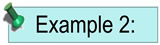Data was collected from 13 students regarding their number extra-curricular activities and their current Grade Point Averages.

Is this a linear regression?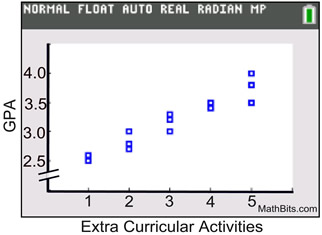The scatter plot appears that the plots are rising from left to right, and are clustered around a possible straight line.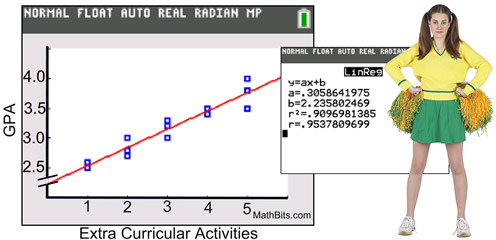The calculator prepared a linear regression
on the data and arrived at the
linear regression equation y = 0.306x + 2.236
(rounded to nearest thousandths).
 Extra-Curr. Activities GPA 1 2.5 1 2.6 2 2.7 2 2.8 2 3.0 3 3.0 3 3.2 3 3.3 4 3.4 4 3.5 5 3.5 5 3.8 5 4.0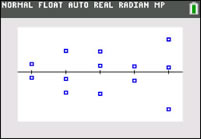Residual plots shows no pattern.
Linear model is appropriate.

What is the slope of the linear regression line, and what does it tell us?
The slope is the "a" value from the calculator ( y = ax + b). The slope is 0.306.
It tells us that the for every additional extra currirular activity, we can predict an increase of 0.306 points on the GPA.

What does the y-intercept represent?
The y-intercept is where x = 0. In this example, x = 0 stands for "no" extra curricular activities.
The y-intercept predicts that a student with no extra curricular activities will have a GPA of 2.236.

What GPA can we predict for a student with 8 extra curricular activities?
This question is asking us to extrapolate, based upon the linear regression equation. Substitute 8 for x: y = 0.306(8) + 2.236. The GPA will be 4.684. The problem, however, is that most schools have 4.0 as an upper limit on GPAs. We may need to place a constraint on the range (no scores above 4.0) to keep our predictions realistic.

If a student has a GPA of 3.35, how many extra curricular activities are they likely to have?
This question is asking us to interpolate, based upon the linear regression equation. Substitute 3.35 for y and solve for x: 3.35 = 0.306x + 2.236. The solution shows that the student will have 3.64 extra curricular activities, whatever that means. Students don't have 64/100 of an activity. We would have to make a decision as to whether we have this answer be interpreted as 3 activities or round it to 4 activities.